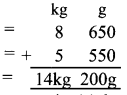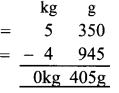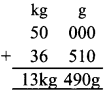# DAV Class 4 Maths Chapter 6 Worksheet 4 Solutions

The DAV Class 4 Maths Book Solutions and DAV Class 4 Maths Chapter 6 Worksheet 4 Solutions of Weight offer comprehensive answers to textbook questions.

## DAV Class 4 Maths Ch 6 WS 4 Solutions

Question 1.
Solve the following word problems.
(a) Two baskets contain 8 kg 650 g and 5 kg 550 g of tomatoes. How much is the total quantity of tomatoes?
Tomatoes in one basket = 8kg 650g
Tomatoes in other basket = 5kg 550g
Total quantity = 14kg 200gThus, total quantity of tomatoes is 14 kg 200 g.

(b) A family consumed 40 kg 800 g flour in the month of December, 25 kg 500 g in the month of January and 38 kg 750 g in the month of February. What was the total consumption of flour for the three months?
Flour consumed in Dec. = 40kg 800g
Flour consumed in Jan. = + 25kg 500g
Flour consumed in Feb. = + 38kg 750g
Total consumption = 105kg 050gThus, total consumption of flour for the three months is 105 kg 50 g.(c) In a ration shop, there was 1250 kg 875 g of wheat in the morning. During the day, 1080 kg 250 g of wheat has sold out. By the evening how much of wheat was left in the shop?
Quantity of wheat in shop = 1250 kg 875 g
Quantity of wheat sold out = – 1080 kg 250 g
Quantity of wheat left = 170kg 625gThus, 170 kg 625 g of wheat was left in the shop.

(d) The weight of one watermelon is 5 kg 350 g and that of another is 4 kg 945 g. Which watermelon is of more quantity and by how much?
As 5 kg 350 g > 4 kg 945 g
wt. of 1st watermelon = 5kg 350g
wt. of 2nd watermelon = 4kg 945gThus, wt. of 1st watermelon is greater than 2nd watermelon by 405 g.

Word Problems:

E.g. A fruit seller had 26 kg 700 g of apples, in his shop. What is the total quantity of fruits in his shop?
Quantity of apples = 26 kg 700 g
Quantity of oranges = 32 kg 570 g
Quantity of pears = 8 kg 910 g
Total quantity = 68 kg 180 gThus, total quantity of fruits in his shop is 68 kg 180 gE.g.
Mohan’s mother used 36 kg 510 g of ghee out of a container of 50 kg of ghee. How much ghee is left in the container?